# Sphere

Here we will learn about spheres, including how to find the volume and surface area of a sphere.

There are also sphere worksheets based on Edexcel, AQA and OCR exam questions, along with further guidance on where to go next if you’re still stuck.

## What is a sphere shape?

A sphere shape is a three dimensional shape where every point of its surface is equidistant (the same distance) from the centre of the sphere. The distance from the centre of a sphere to its surface is called the radius. A sphere has no vertices.

A hemisphere is half a sphere.

### What is a sphere shape?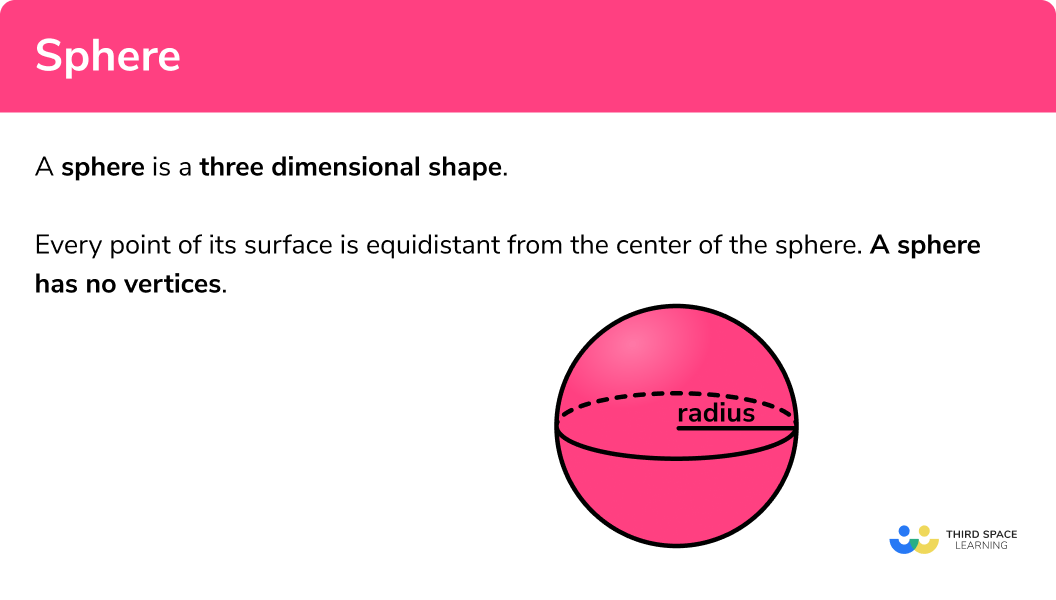### Volume of a sphere shape

The volume of a sphere is the amount of space there is inside a sphere.

The formula for the volume of a sphere is:

\text{Volume}=\frac{4}{3}\pi{r}^3

E.g. Find the volume of the sphere

This sphere has a radius of 7 \; cm .

\begin{aligned} \text{Volume}&=\frac{4}{3} \pi r^3 \\\\ &= \frac{4}{3} \times \pi \times 7^3\\\\ &=\frac{1372}{3}\pi\\\\ &=1436.755... \\\\ &=1440 \ cm^3 \ \text{(3sf)} \end{aligned}

Step-by-step guide: Volume of a sphere

### Surface area of a sphere shape

The surface area of a sphere is the area which covers the outer surface of a sphere.

The formula for the surface area of a sphere is:

\text{Surface Area}=4 \pi r^2

E.g. Find the surface area of the sphere

This sphere has a radius of 7 \; cm .

\begin{aligned} \text{Surface area}&=4\pi r^2\\\\ &=4 \times \pi \times 7^2\\\\ &=196\pi\\\\ &=615.752...\\\\ &=616 \ cm^2 \ \text{(to 3 sf)} \end{aligned}

Step-by-step guide: Surface area of a sphere

## How to calculate the volume or surface area of a sphere

In order to calculate the volume of a sphere or the surface area of a sphere:

1. Write down the formula.
2. Substitute the given values.
3. Work out the calculation

### How to calculate the volume or surface area of a sphere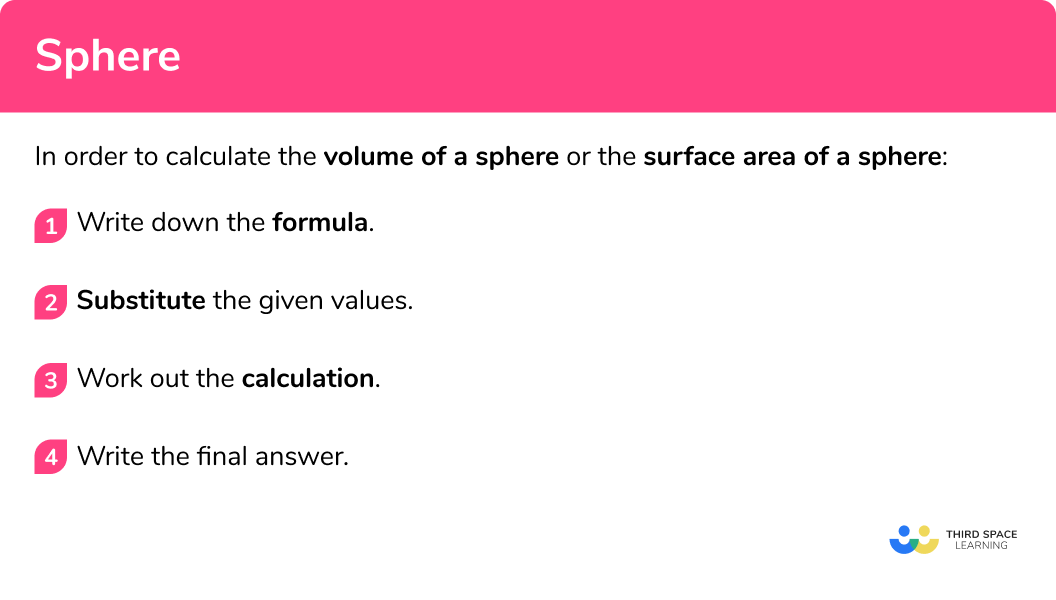## Sphere shape examples

### Example 1: volume of a sphere

Calculate the volume of a sphere of radius 12 \; cm. Give your answer to 3 significant figures.

1. Write down the formula for the volume of a sphere.

\text{Volume}=\frac{4}{3} \pi r^3

2Substitute the given values into the formula.

V=\frac{4}{3} \times \pi \times 12^3

3Complete the calculation.

\begin{aligned} &=2304\pi \\\\ &=7238.229... \end{aligned}

4Write the final answer, including the units.

The radius is given in centimetres so the volume will be cubic centimetres.

The volume of the sphere is 7240 \; cm^3 (3 sf) .

### Example 2: volume of a sphere, given the diameter

Calculate the volume of a sphere with a diameter of 30 \; cm . Write your answer in terms of \pi .

Write down the formula for the volume of a sphere.

Substitute the given values into the formula.

Complete the calculation.

Write the final answer, including the units.

### Example 3: volume of a hemisphere

Calculate the volume of a hemisphere of radius 4 \; cm . Write your answer to 2 decimal places.

Write down the formula for the volume of a sphere.

Substitute the given values into the formula.

Complete the calculation.

Write the final answer, including the units.

### Example 4: surface area of a sphere

Calculate the surface area of a sphere with radius 7 \; cm . Write your answer to 1 decimal place.

Write down the formula for the surface area of a sphere.

Substitute the given values into the formula.

Complete the calculation.

Write the final answer, including the units.

### Example 5: surface area of a sphere given the diameter – in terms of π

Find the surface area of a sphere with the diameter of 20 \; cm. Leave your answer in terms of \pi .

Write down the formula for the surface area of a sphere.

Substitute the given values into the formula.

Complete the calculation.

Write the final answer, including the units.

### Example 6: total surface area of a hemisphere

Find the total surface area of a hemisphere with a radius 4 \; cm. Write your answer to 3  significant figures.

Write down the formula for the surface area of a sphere.

Substitute the given values into the formula.

Complete the calculation.

Write the final answer, including the units.

### Common misconceptions

• Using the correct formula

There are several formulas that can be used, so we need to match the correct formula to the correct context.

• Rounding

It is important to not round the answer until the end of the calculation. This will mean your final answer is accurate.

• Make sure you have the correct units

For area we use square units such as cm^2, m^2, km^2.

For volume we use cube units such as cm^3, m^3, km^3.

• Using the radius or the diameter

It is a common error to mix up radius and diameter. Remember the radius is half of the diameter.

### Practice sphere shape questions

1. Find the volume of a sphere with radius 4.3 \; cm. Write your answer to 2 decimal places.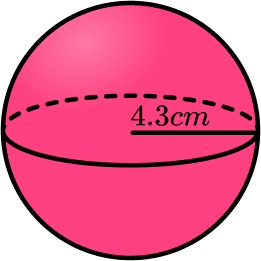333.04 \; cm^3330 \; m^358.09 \; cm^3232.35 \; cm^3We are finding the volume of a sphere so we substitute the value of the radius r=4.3 into the formula V=\frac{4}{3}\pi{r}^{3}

\begin{aligned} \text{Volume}&=\frac{4}{3} \pi r^3 \\\\ &= \frac{4}{3} \times \pi \times 12^3\\\\ &=333.0381428… \\\\ &=333.04\text{cm}^{3} \ \text{(2dp)} \end{aligned}

2. Find the volume of a sphere with radius 18 \; cm. Leave your answer in terms of \pi .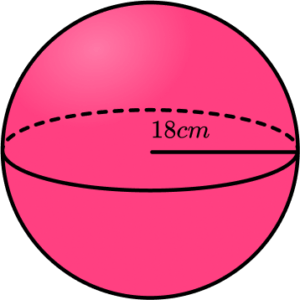432\pi \; cm^31944\pi \; m^37776\pi \; cm^35832\pi \; cm^3We are finding the volume of a sphere so we substitute the value of the radius into the formula V=\frac{4}{3}\pi{r}^{3} . As the diameter is twice the length of the radius, r=36 \div 2=18 \;cm.

\begin{aligned} \text{Volume}&=\frac{4}{3} \pi r^3 \\\\ &= \frac{4}{3} \times \pi \times 18^3\\\\ &=7776\pi \\\\ &=7776\pi \; cm^3 \end{aligned}

3. Find the volume of a hemisphere with radius 6.2 \; cm. Write your answer to 1 decimal place.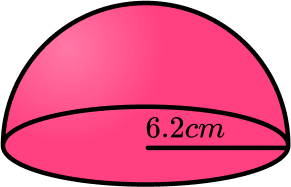362.3 \; cm^3998.3 \; m^3499.2 \; cm^3120.8 \; cm^3We are finding the volume of a hemisphere, so we need to calculate half of the volume of a sphere using the radius r=6.2 \; cm, and the formula: V=(\frac{4}{3}\pi{r}^3)\div{2}.

\begin{aligned} V&=(\frac{4}{3}\pi{r}^3)\div{2}\\\\ &=(\frac{4}{3}\times\pi\times{6.2}^3)\div{2}\\\\ &=499.152996…\\\\ &=499.2\text{ cm}^3 \end{aligned}

4. Find the surface area of a sphere with radius 4.6 \; cm. Give your answer to 2 decimal places.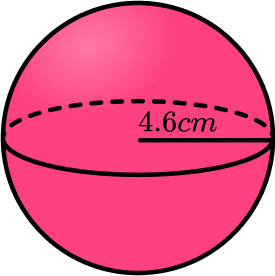270\text{ cm}^2407.72\text{ cm}^266.48\text{ cm}^2265.90\text{ cm}^2We are finding the surface area of a sphere, so we substitute the value of the radius r=4.6 into the formula SA=4\pi r^2.

\begin{aligned} \text{Surface area}&=4\pi r^2\\\\ &=4 \times \pi \times 4.6^2\\\\ &=265.9044022…\\\\ &=265.90 \text{ cm}^2 \ \text{(2dp)} \end{aligned}

5. Find the surface area of a sphere with a diameter of 16 \; mm. Leave your answer in terms of \pi.

1024\pi\text{ mm}^264\pi\text{ mm}^2256\pi \text{ mm}^22144\pi\text{ mm}^2We are finding the surface area of a sphere, so we need to calculate the value of the radius using the diameter, then substitute the value of the radius into the formula for the surface area.
r=16 \div 2=8 \; mm

\begin{aligned} \text{Surface area}&=4\pi r^2\\\\ &=4 \times \pi \times 8^2\\\\ &=256\pi \ \text{mm}^2 \end{aligned}

6. Find the surface of a hemisphere with radius 7.8 \; cm. Give your answer to 1 decimal place.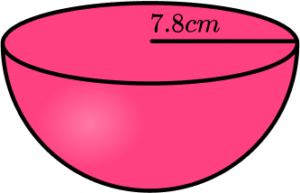573.4 \text{ cm}^2764.5 \text{ cm}^2191.1 \text{ cm}^2993.9 \text{ cm}^2We need to find the curved surface area by finding half of the surface area of a sphere. Then we add on the area of the circular top.

\begin{aligned} \text{Total surface area}&=3\pi r^2\\\\ &=3 \times \pi \times{7.8}^2\\\\ &=573.4034911…\\\\ &=573.4 \text{ cm}^2 \ \text{(1dp)} \end{aligned}

### Sphere shape GCSE questions

\text{Surface area of a sphere}=4 \pi r^2

1. Here is a sphere with a radius 8.4 \; cm.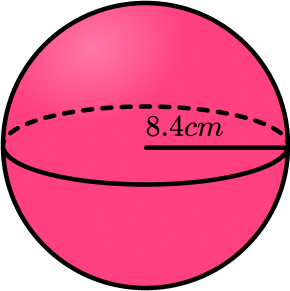Calculate the volume of the sphere.

(2 marks)

V=\frac{4}{3} \times \pi \times 8.4^3

(1)

2482.71271…=2482.7 \; cm^3

(1)

2. A tennis ball has a radius of 3.4 \; cm. Each tennis ball is covered in felt. Calculate the area of the felt required to cover the ball to the nearest square millimetre.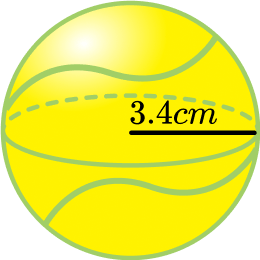(4 marks)

3.4 \; cm=34 \; mm

(1)

SA=4\times \pi \times{34}^2

(1)

14526.72443…

(1)

14527 \; mm^2

(1)

3. A container is a hemisphere with a diameter of 70 \; cm.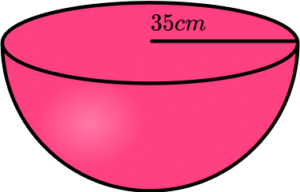Sand fills the container at a rate of 5000 \; cm^3 per minute.

How long does it take to fill the container?

(3 marks)

(\frac{4}{3} \times \pi \times 35^3) \div{2} = 89797.19002…

(1)

89797.19002… \div{5000}=17.959438…

(1)

17.959438… = 18 minutes

(1)

4. A sphere has a surface area of 1000 \; m^2.

Calculate its volume.

(3 marks)

r=\sqrt{\frac{1000}{4\pi}}=8.920620581…

(1)

\frac{4}{3}\times \pi \times8.920620581^3=2973.540194…

(1)

2973.540194…=2970 \; m^3 \; (3sf)

(1)

## Learning checklist

You have now learned how to:

• Find the volume of a sphere
• Find the surface area of a sphere
• How to solve problems involving spheres

## Still stuck?

Prepare your KS4 students for maths GCSEs success with Third Space Learning. Weekly online one to one GCSE maths revision lessons delivered by expert maths tutors.

Find out more about our GCSE maths tuition programme.